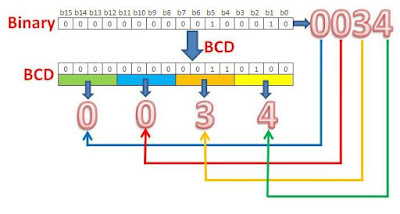# What is a BIT...? And BCD

What is a BIT:

As we know a bit is the smallest unit of data in a computer system

A bit can either be a 12 or a 02

This means that with one single bit we can only represent only 2 different values

12 = 110 and 02 = 010Representing Text, Pictures etc…
Text, pictures and videos are also stored in a computer system using a sequence of binary digits

Using one single bit is not enough due to its small size

If we want to store a large quantity of data many more bits are required.

Combining a number of Bits:
Each time a bit is combined with another bit, more different combinations can be stored.

If we will be using two bits to store data, there are a total of 22= 4 different combinations

Hence if we have 4 bits we would have 24= 16 different combinationsWorking:

With the following number of bits how many combinations could be created?
1.5 bits
2.3 bits
3.6 bits
4.8 bits

1.5 bits = 25 à 32 combinations

2.3 bits = 23 à 8 combinations

3.6 bits = 26 à 64 combinations

4.8 bits = 28 à 256 combinations

In the Early Days

When computers were first introduced they were simply used for performing calculations
After a while scientists realized that computers can also be used to store and edit text in different ways

The problem was that a computer could only understand binary (1s & 0s)

BCD(binary coded decimal)

Very early memory codes.
Each decimal number converted to binary equivalent individually.
For example 39=0011 1001
Each decimal digit is converted individually to four bit binary
4 bits=>16 different characters.
Only sufficient to represent 10 numbers.
6 combinations are redundant.6 bit BCD

To represent other characters on keyboard 6 bit BCD is used.
6 bits=> 64 different characters.
Sufficient for 26 alphabet+28 special characters+ 10 digits.
Can only present small letters.

EBCDIC

64 different BCD codes are not sufficient for all the English letters upper and lower.
So Extended Binary coded decimal information interchange is used.
Its 8 bit code=>256 different symbols.